# RD Sharma Solutions for Class 6 Maths Chapter 4: Operations on Whole Numbers Exercise 4.4

## RD Sharma Solutions for Class 6 Maths Exercise 4.4 PDF

RD Sharma Solutions consists of problems solved by subject experts after conducting wide research on each concept. They mainly focus on the concepts which are important for the final exam. The methods of solving problems are based on the CBSE syllabus and guidelines. The step-wise detailed structure of the solutions improves knowledge about the concepts among students. PDFs of solutions can be downloaded from BYJU’S website, which are important for exam preparation. RD Sharma Solutions for Class 6 Maths Chapter 4 Operations on Whole Numbers Exercise 4.4 are provided here.

## RD Sharma Solutions for Class 6 Maths Chapter 4: Operations on Whole Numbers Exercise 4.4 Download the PDF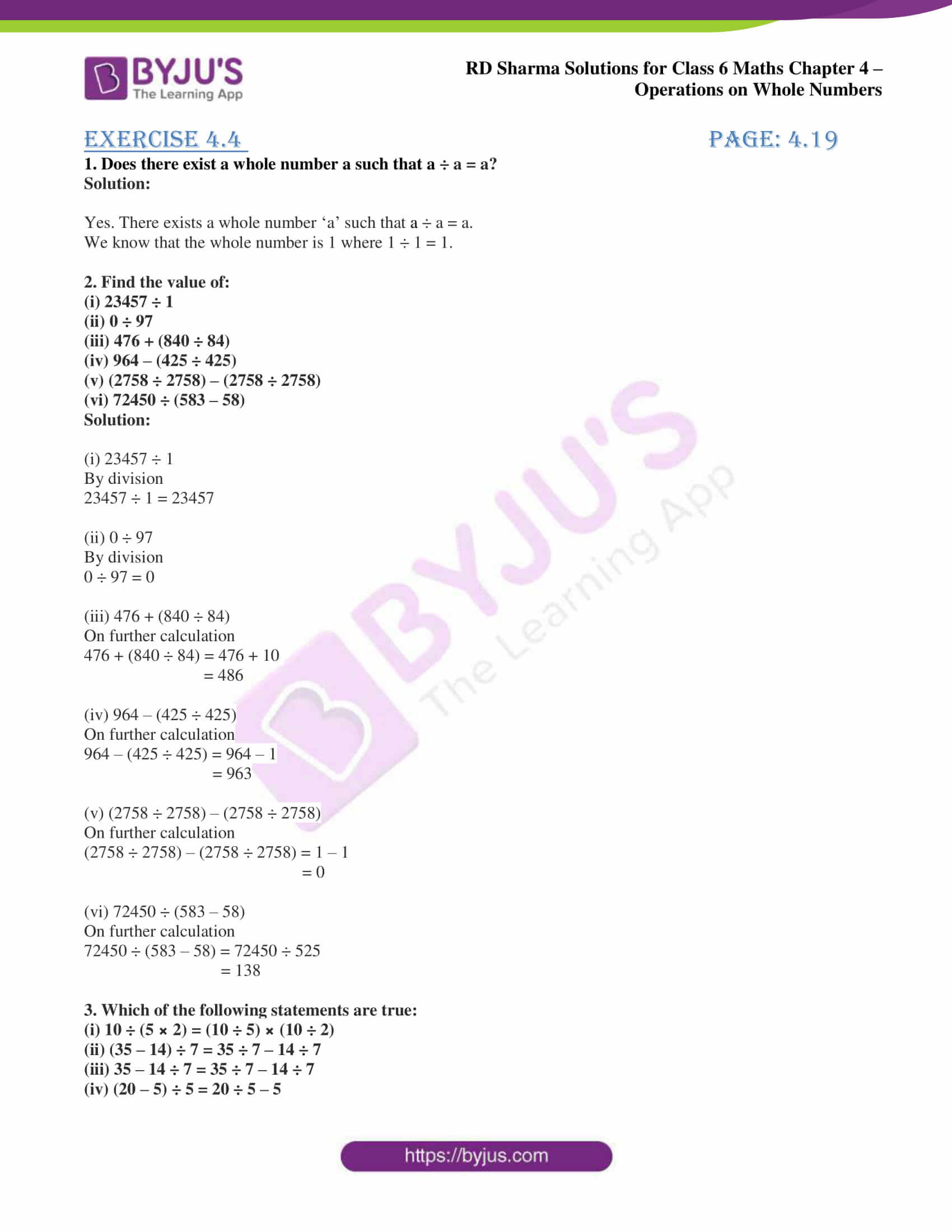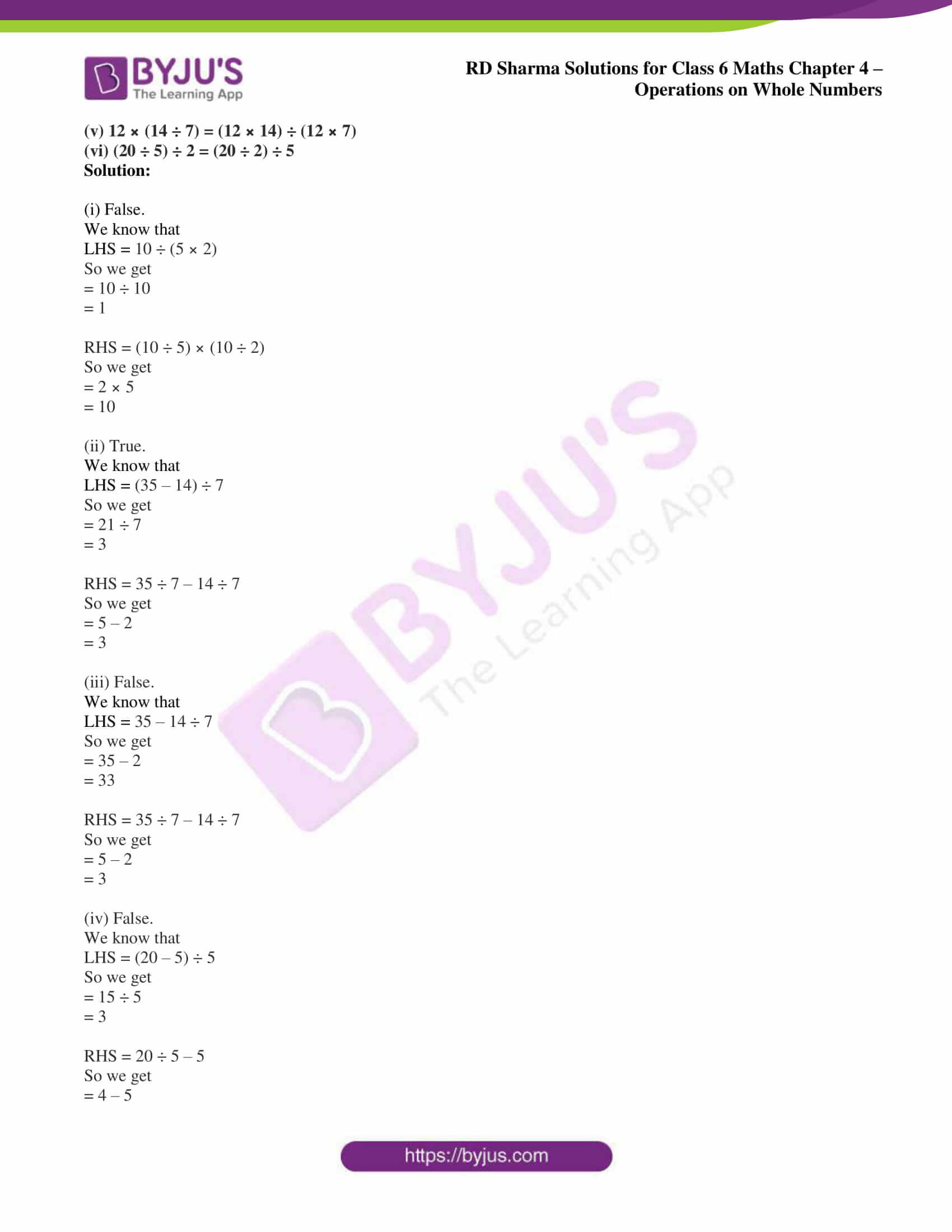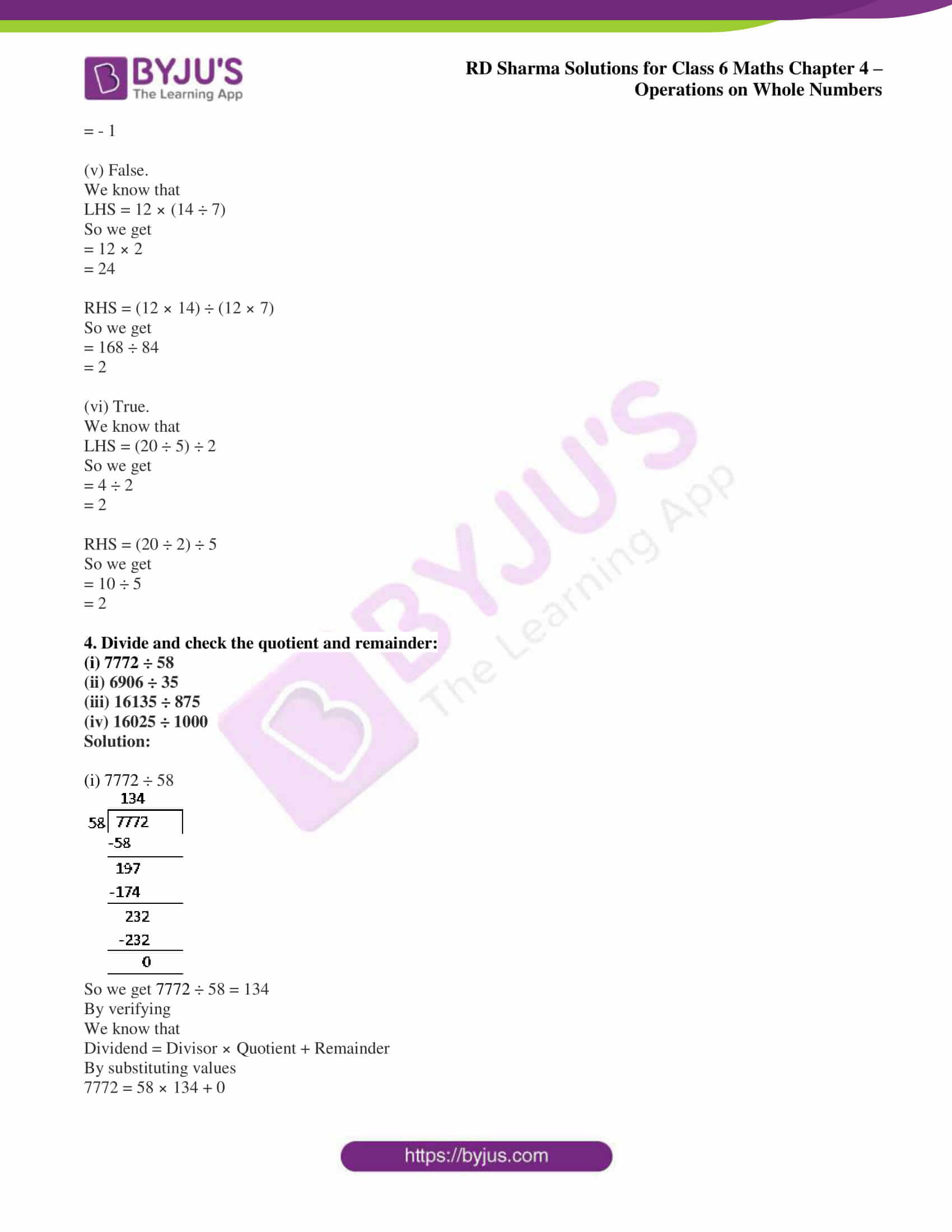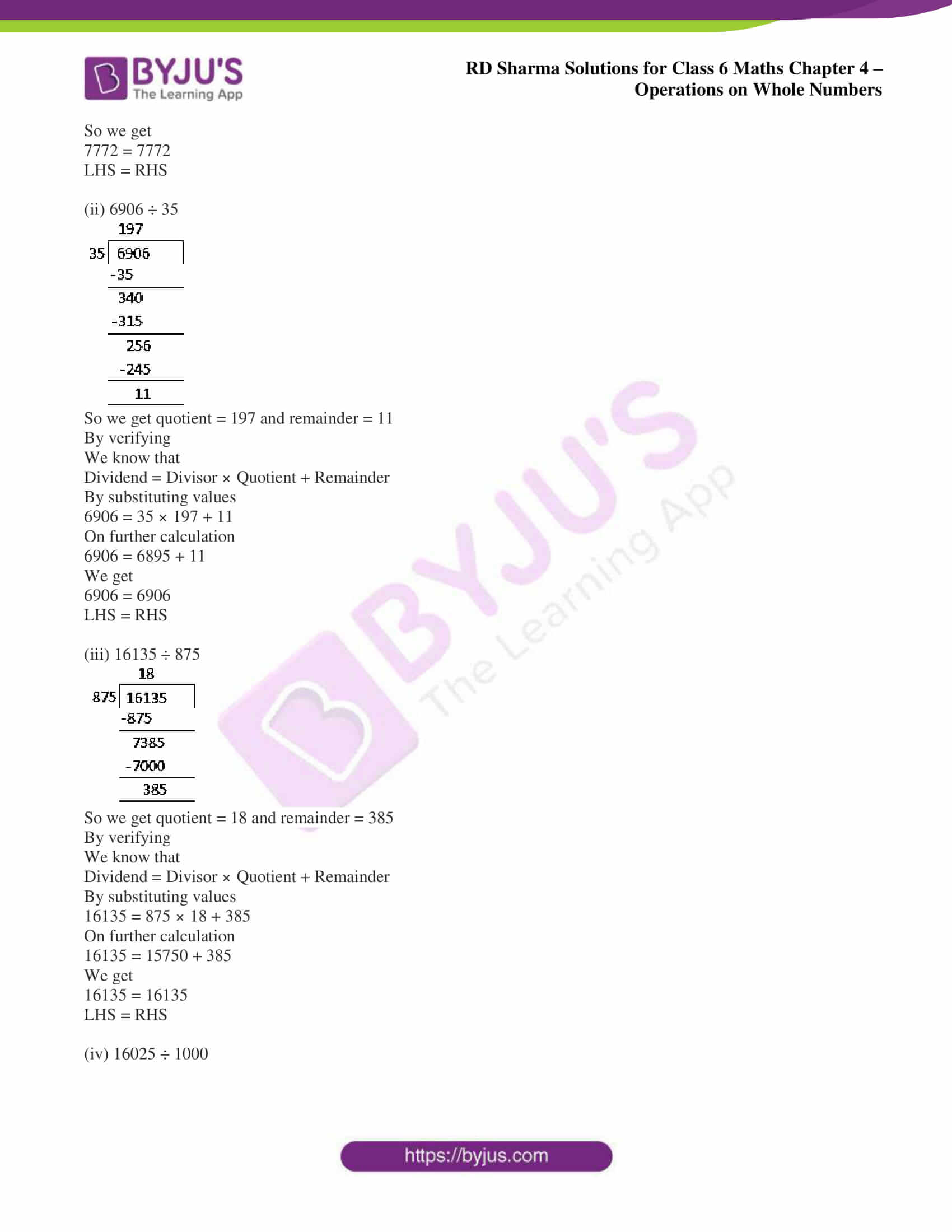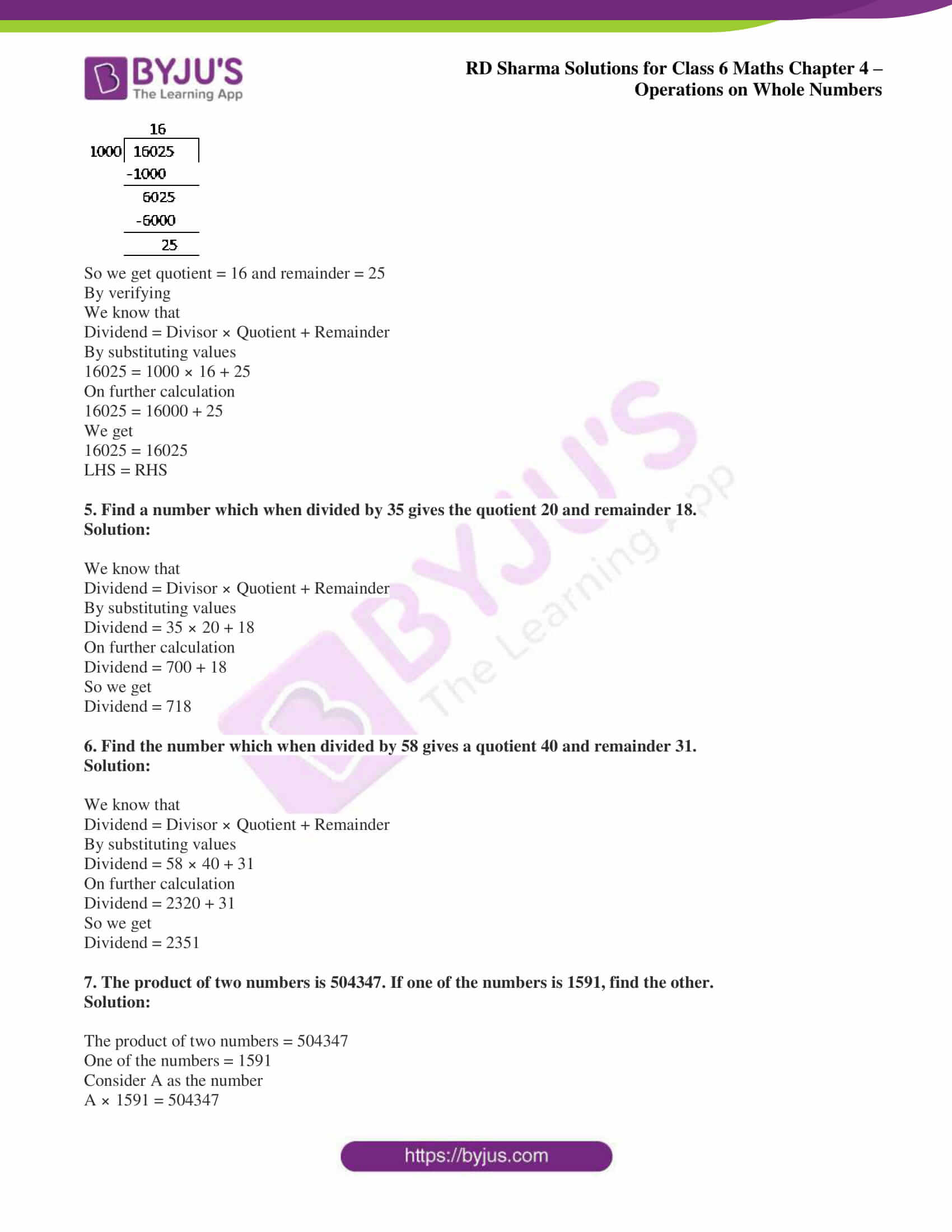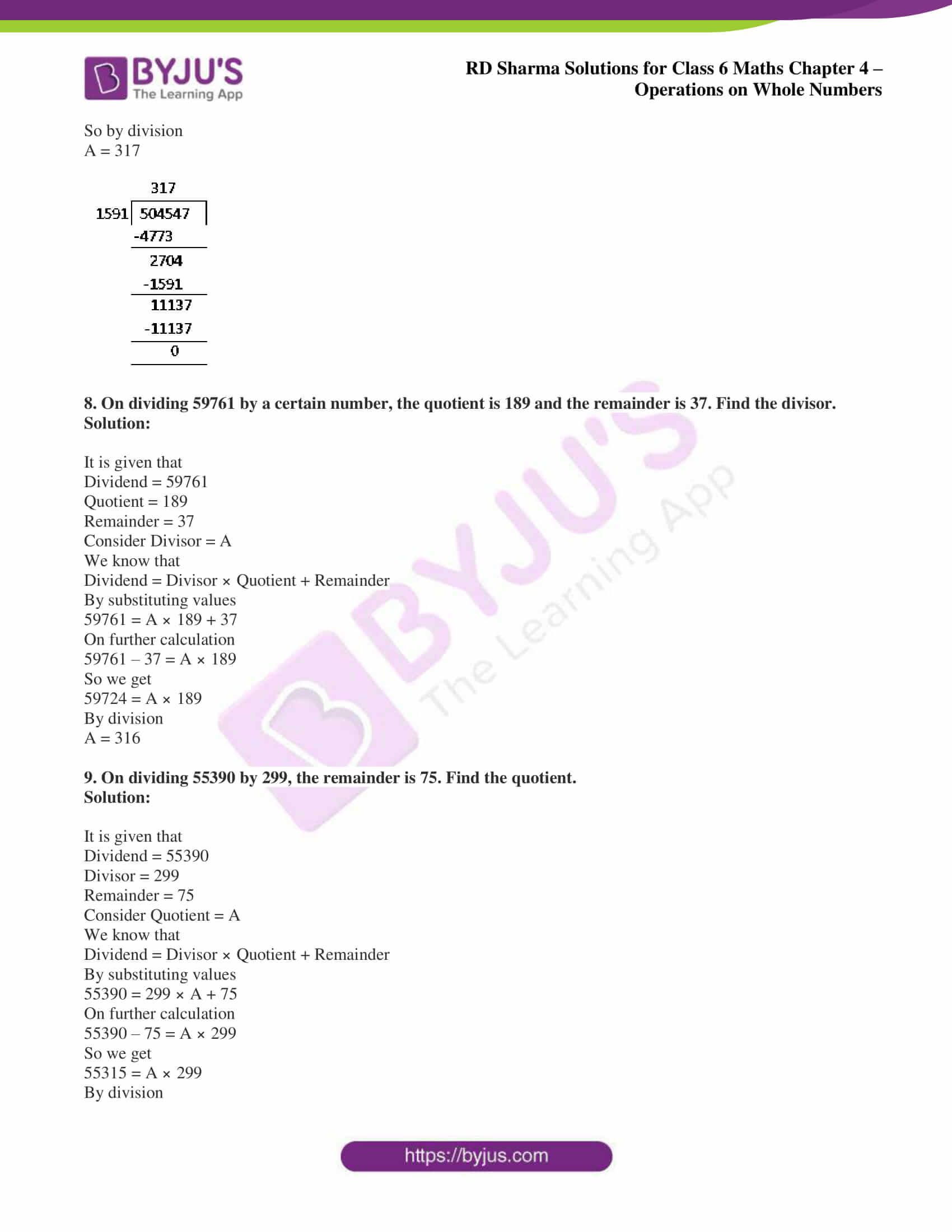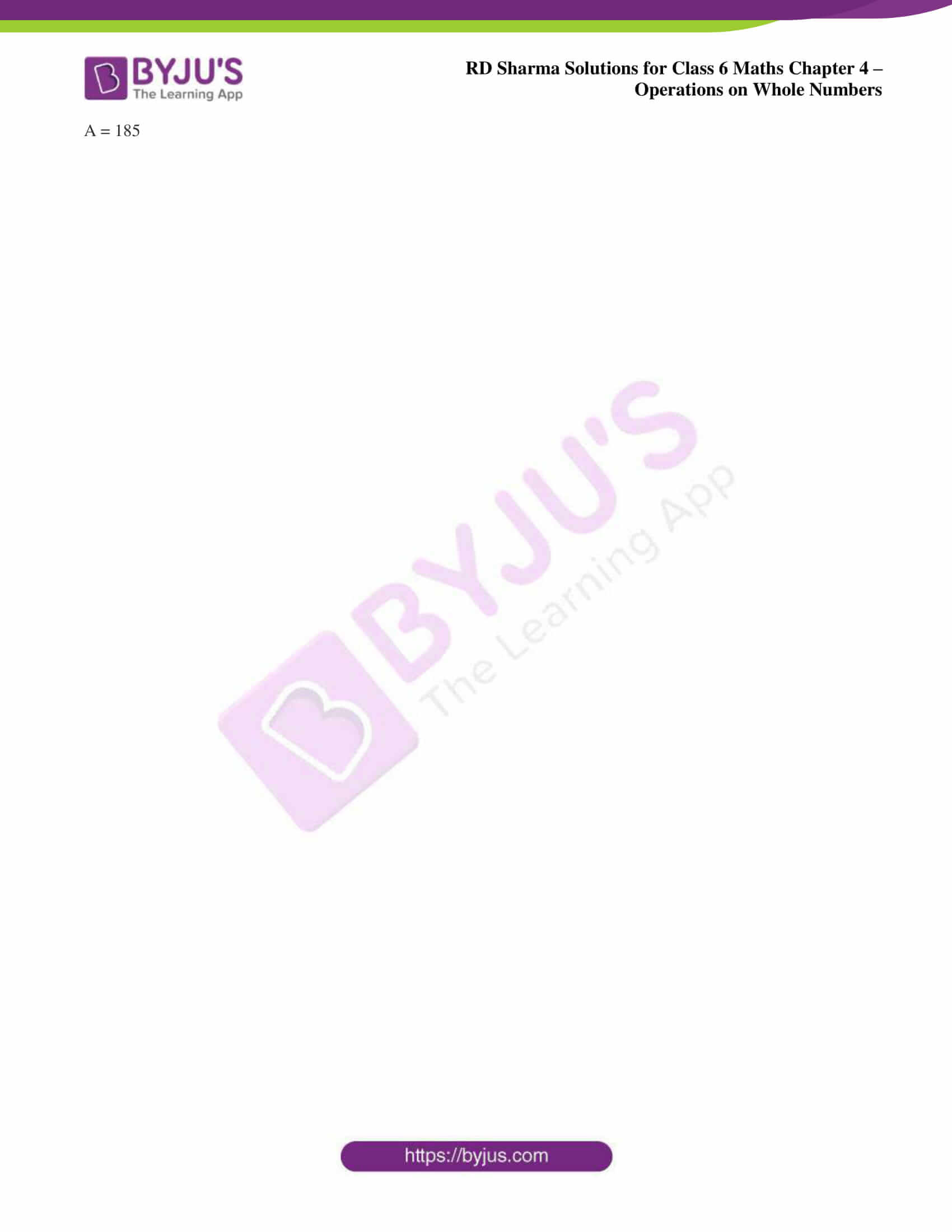## Access answers to Maths RD Sharma Solutions for Class 6 Chapter 4: Operations on Whole Numbers Exercise 4.4

1. Does there exist a whole number a such that a ÷ a = a?

Solution:

Yes. There exists a whole number ‘a’ such that a ÷ a = a.

We know that the whole number is 1 where 1 ÷ 1 = 1.

2. Find the value of:

(i) 23457 ÷ 1

(ii) 0 ÷ 97

(iii) 476 + (840 ÷ 84)

(iv) 964 – (425 ÷ 425)

(v) (2758 ÷ 2758) – (2758 ÷ 2758)

(vi) 72450 ÷ (583 – 58)

Solution:

(i) 23457 ÷ 1

By division

23457 ÷ 1 = 23457

(ii) 0 ÷ 97

By division

0 ÷ 97 = 0

(iii) 476 + (840 ÷ 84)

On further calculation

476 + (840 ÷ 84) = 476 + 10

= 486

(iv) 964 – (425 ÷ 425)

On further calculation

964 – (425 ÷ 425) = 964 – 1

= 963

(v) (2758 ÷ 2758) – (2758 ÷ 2758)

On further calculation

(2758 ÷ 2758) – (2758 ÷ 2758) = 1 – 1

= 0

(vi) 72450 ÷ (583 – 58)

On further calculation

72450 ÷ (583 – 58) = 72450 ÷ 525

= 138

3. Which of the following statements are true:

(i) 10 ÷ (5 × 2) = (10 ÷ 5) × (10 ÷ 2)

(ii) (35 – 14) ÷ 7 = 35 ÷ 7 – 14 ÷ 7

(iii) 35 – 14 ÷ 7 = 35 ÷ 7 – 14 ÷ 7

(iv) (20 – 5) ÷ 5 = 20 ÷ 5 – 5

(v) 12 × (14 ÷ 7) = (12 × 14) ÷ (12 × 7)

(vi) (20 ÷ 5) ÷ 2 = (20 ÷ 2) ÷ 5

Solution:

(i) False.

We know that

LHS = 10 ÷ (5 × 2)

So we get

= 10 ÷ 10

= 1

RHS = (10 ÷ 5) × (10 ÷ 2)

So we get

= 2 × 5

= 10

(ii) True.

We know that

LHS = (35 – 14) ÷ 7

So we get

= 21 ÷ 7

= 3

RHS = 35 ÷ 7 – 14 ÷ 7

So we get

= 5 – 2

= 3

(iii) False.

We know that

LHS = 35 – 14 ÷ 7

So we get

= 35 – 2

= 33

RHS = 35 ÷ 7 – 14 ÷ 7

So we get

= 5 – 2

= 3

(iv) False.

We know that

LHS = (20 – 5) ÷ 5

So we get

= 15 ÷ 5

= 3

RHS = 20 ÷ 5 – 5

So we get

= 4 – 5

= – 1

(v) False.

We know that

LHS = 12 × (14 ÷ 7)

So we get

= 12 × 2

= 24

RHS = (12 × 14) ÷ (12 × 7)

So we get

= 168 ÷ 84

= 2

(vi) True.

We know that

LHS = (20 ÷ 5) ÷ 2

So we get

= 4 ÷ 2

= 2

RHS = (20 ÷ 2) ÷ 5

So we get

= 10 ÷ 5

= 2

4. Divide and check the quotient and remainder:

(i) 7772 ÷ 58

(ii) 6906 ÷ 35

(iii) 16135 ÷ 875

(iv) 16025 ÷ 1000

Solution:

(i) 7772 ÷ 58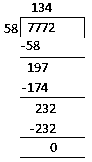So we get 7772 ÷ 58 = 134

By verifying

We know that

Dividend = Divisor × Quotient + Remainder

By substituting values

7772 = 58 × 134 + 0

So we get

7772 = 7772

LHS = RHS

(ii) 6906 ÷ 35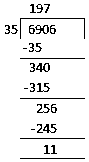So we get quotient = 197 and remainder = 11

By verifying

We know that

Dividend = Divisor × Quotient + Remainder

By substituting values

6906 = 35 × 197 + 11

On further calculation

6906 = 6895 + 11

We get

6906 = 6906

LHS = RHS

(iii) 16135 ÷ 875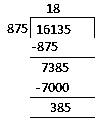So we get quotient = 18 and remainder = 385

By verifying

We know that

Dividend = Divisor × Quotient + Remainder

By substituting values

16135 = 875 × 18 + 385

On further calculation

16135 = 15750 + 385

We get

16135 = 16135

LHS = RHS

(iv) 16025 ÷ 1000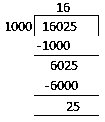So we get quotient = 16 and remainder = 25

By verifying

We know that

Dividend = Divisor × Quotient + Remainder

By substituting values

16025 = 1000 × 16 + 25

On further calculation

16025 = 16000 + 25

We get

16025 = 16025

LHS = RHS

5. Find a number which when divided by 35 gives the quotient 20 and remainder 18.

Solution:

We know that

Dividend = Divisor × Quotient + Remainder

By substituting values

Dividend = 35 × 20 + 18

On further calculation

Dividend = 700 + 18

So we get

Dividend = 718

6. Find the number which when divided by 58 gives a quotient 40 and remainder 31.

Solution:

We know that

Dividend = Divisor × Quotient + Remainder

By substituting values

Dividend = 58 × 40 + 31

On further calculation

Dividend = 2320 + 31

So we get

Dividend = 2351

7. The product of two numbers is 504347. If one of the numbers is 1591, find the other.

Solution:

The product of two numbers = 504347

One of the numbers = 1591

Consider A as the number

A × 1591 = 504347

So by division

A = 317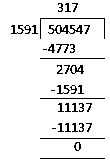8. On dividing 59761 by a certain number, the quotient is 189 and the remainder is 37. Find the divisor.

Solution:

It is given that

Dividend = 59761

Quotient = 189

Remainder = 37

Consider Divisor = A

We know that

Dividend = Divisor × Quotient + Remainder

By substituting values

59761 = A × 189 + 37

On further calculation

59761 – 37 = A × 189

So we get

59724 = A × 189

By division

A = 316

9. On dividing 55390 by 299, the remainder is 75. Find the quotient.

Solution:

It is given that

Dividend = 55390

Divisor = 299

Remainder = 75

Consider Quotient = A

We know that

Dividend = Divisor × Quotient + Remainder

By substituting values

55390 = 299 × A + 75

On further calculation

55390 – 75 = A × 299

So we get

55315 = A × 299

By division

A = 185

### RD Sharma Solutions for Class 6 Maths Chapter 4 – Operations on Whole Numbers Exercise 4.4

RD Sharma Solutions Class 6 Maths Chapter 4 Operations on Whole Numbers Exercise 4.4 help students gain knowledge about division and the important properties which are used in solving exercise-wise problems.

### Key Features of RD Sharma Solutions for Class 6 Maths Chapter 4: Operations on Whole Numbers Exercise 4.4

• Problem-solving and logical thinking abilities are improved among students.
• The solutions help students solve problems more accurately and in an efficient manner.
• They aid students in grasping the important methods which are used in solving problems based on the CBSE guidelines.
• The solutions can be referred to while solving exercise-wise problems in the RD Sharma textbook for the latest syllabus.